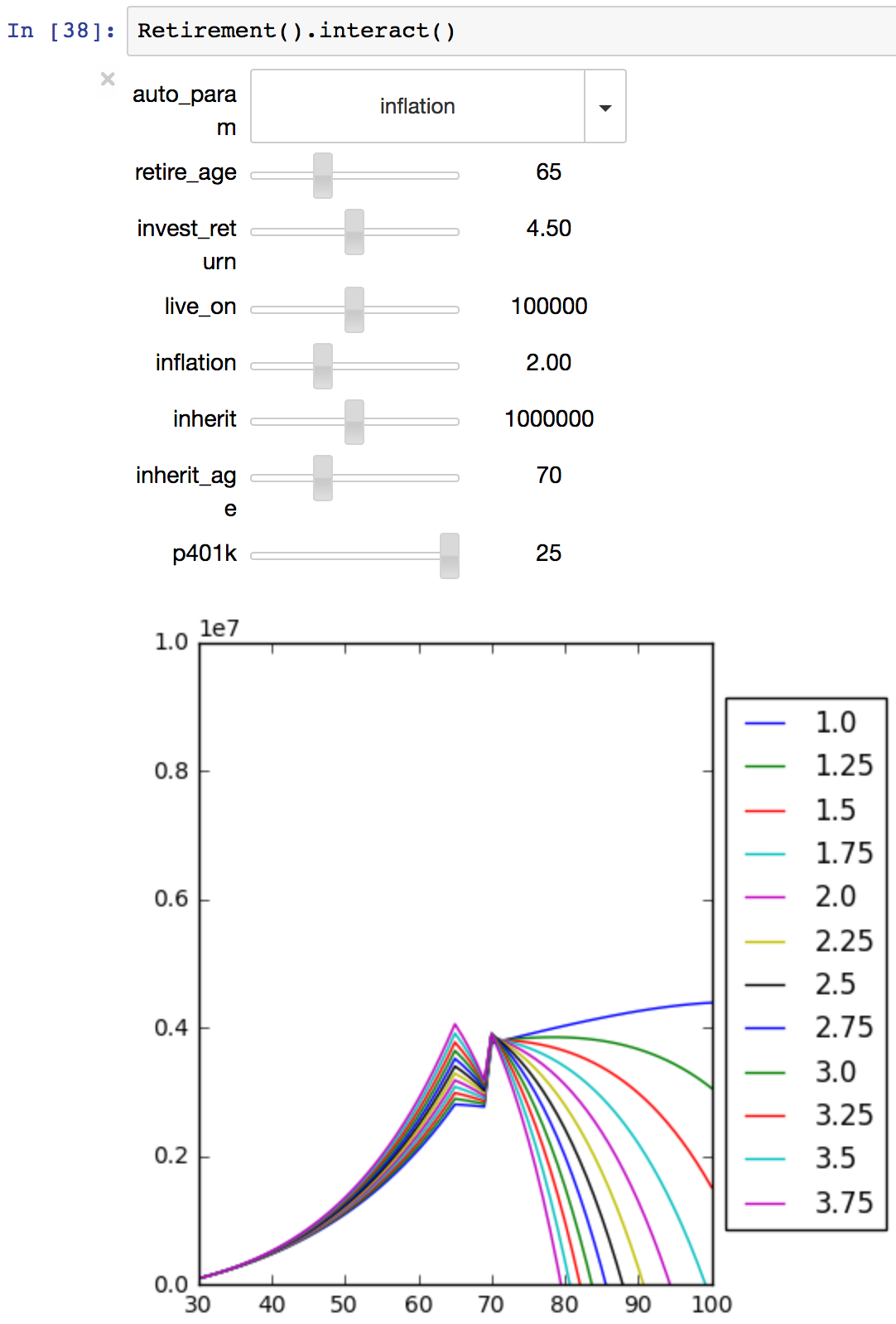# Multi-parameter Jupyter notebook interaction

I’m working on figuring out retirement scenarios. I wasn’t satified with the usual online calculators. I made a spreadsheet, but it was hard to see how the different variables affected the outcome. Aha! This sounds like a good use for a Jupyter Notebok!

Using widgets, I could make a cool graph with sliders for controlling the variables, and affecting the result. Nice.

But there was a way to make the relationship between the variables and the outcome more apparent: choose one of the variables, and plot its multiple values on a single graph. And of course, I took it one step further, so that I could declare my parameters, and have the widgets, including the selection of the variable to auto-slide, generated automatically.

I’m pleased with the result, even if it’s a little rough. You can download retirement.ipynb to try it yourself.

The general notion of a declarative multi-parameter model with an auto-slider is contained in a class:

```%pylab --no-import-all inlinefrom collections import namedtuplefrom ipywidgets import interact, IntSlider, FloatSliderclass Param(namedtuple('Param', "default, range")):    """    A parameter for `Model`.    """    def make_widget(self):        """Create a widget for a parameter."""        is_float = isinstance(self.default, float)        is_float = is_float or any(isinstance(v, float) for v in self.range)        wtype = FloatSlider if is_float else IntSlider        return wtype(            value=self.default,            min=self.range, max=self.range, step=self.range,
continuous_update=True,        )class Model:    """    A multi-parameter model.    """    output_limit = None    num_auto = 7
def _show_it(self, auto_param, **kw):        if auto_param == 'None':            plt.plot(self.inputs, self.run(self.inputs, **kw))        else:            autop = self.params[auto_param]            auto_values = np.arange(*autop.range)            if len(auto_values) > self.num_auto:                lo, hi = autop.range[:2]                auto_values = np.arange(lo, hi, (hi-lo)/self.num_auto)            for auto_val in auto_values:                kw[auto_param] = auto_val                output = self.run(self.inputs, **kw)                plt.plot(self.inputs, output, label=str(auto_val))            plt.legend(loc='center left', bbox_to_anchor=(1, 0.5))        if self.output_limit is not None:            plt.ylim(*self.output_limit)    def interact(self):        widgets = {            name:p.make_widget() for name, p in self.params.items()        }        param_names = ['None'] + sorted(self.params)        interact(self._show_it, auto_param=param_names, **widgets)```

To make a model, derive a class from Model. Define a dict called params as a class attribute. Each parameter has a default value, and a range of values it can take, expressed (min, max, step):

`class Retirement(Model):    params = dict(        invest_return=Param(3, (1.0, 8.0, 0.5)),        p401k=Param(10, (0, 25, 1)),        retire_age=Param(65, (60, 75, 1)),        live_on=Param(100000, (50000, 150000, 10000)),        inflation=Param(2.0, (1.0, 4.0, 0.25)),        inherit=Param(1000000, (0, 2000000, 200000)),        inherit_age=Param(70, (60, 90, 5)),    )`

Your class can also have some constants:

`start_savings = 100000salary = 100000socsec = 10000`

Define the inputs to the graph (the x values), and the range of the output (the y values):

`inputs = np.arange(30, 101)output_limit = (0, 10000000)`

Finally, define a run method that calculates the output from the inputs. It takes the inputs as an argument, and also has a keyword argument for each parameter you defined:

```def run(self, inputs,
invest_return, p401k, retire_age, live_on,    inflation, inherit, inherit_age):    for year, age in enumerate(inputs):        if year == 0:            yearly_money = [self.start_savings]            continue
inflation_factor = (1 + inflation/100)**year        money = yearly_money[-1]        money = money*(1+(invest_return/100))        if age == inherit_age:            money += inherit        if age <= retire_age:            money += self.salary * inflation_factor *(p401k/100)        else:            money += self.socsec            money -= live_on * inflation_factor        yearly_money.append(money)    return np.array(yearly_money)```

To run the model, just instantiate it and call interact():

`Retirement().interact()`

You’ll get widgets and a graph like this:• The sliders are a mess: if you make too many parameters, the slider and the graph don’t fit on the screen.
• The values chosen for the auto parameter are not “nice”, like tick marks on a graph are nice.
• It’d be cool to be able to auto-slide two parameters at once.
• The code isn’t packaged in a way people can easily re-use.

I thought about fixing a few of these things, but I likely won’t get to them. The code is here in this blog post or in the notebook file if you want it. Ideas welcome about how to make improvements.

BTW: my retirement plans are not based on inheriting a million dollars when I am 70, but it’s easy to add parameters to this model, and it’s fun to play with...Nice. Can you splice the corresponding blog post right into the notebook? :)Ned - I wish you success in achieving early retirement!
(I'm also certain you'll continue coding :)